# Encrypting and Decrypting MQTT Message Payloads

Most MQTT implementations use SSL by default for data security.

However SSL is a link encryption method and doesn’t secure the payload end to end unless SSL is applied to all links.

In addition if the data is stored at the destination it will be unencrypted unless it is encrypted before storage.

As an alternative to using SSL encryption for MQTT data you can instead  encrypt the MQTT payload.

Note: Although this tutorial deals with MQTT it also applied to email and any messaging system that has multiple links.

• No broker set up required
• End to end encryption

• Need to develop your own key exchange procedure.

### Node-Red Implementation

There are a variety of encryption nodes to choose from the most popular being the  node-red-contrib-crypto-js module.

This consists of 4 nodes:

• Encrypt and Decrypt Nodes:
• Digest Node
• HMAC (Hash-based Message Authentication Code) Node
• Encode and Decode

The Encrypt and Decrypt Nodes support the following encryption algorithms:

• AES
• DES
• RC4
• Rabbit
• TripleDES

AES (Advanced Encryption Standard) is currently considered the most secure and the one most commonly used.

AES is a block cipher meaning that it encrypts data in blocks for AES each block is 16 bytes (!28 bits).

If the payload is not an even number of blocks then the last block needs to be padded to make it 16 bytes.

AES uses an encryption key of 128,192 or 256 bits.256 is the most secure.

There are different ways of using the key which are called modes of operation. AES has the following modes:

• Electronic Code Book (ECB)
• Cipher Block Chaining (CBC)
• Counter (CTR)
• Cipher Feed Back (CFB)
• Output Feed Back (OFB)
• Galois Counter Mode(GCM)

There is a very good description of these modes of operation here.

### Encrypting and Decrypting Using CFB (Cipher Feed Back)

This mode requires a 16 byte initialising vector (IV) as well as a key.

Depending on the node/code base you are using the IV may be generated for you.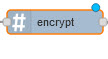Likewise the secret key may also be auto generated from a pass phrase as shown below with the encrypt node for the crypto.js package: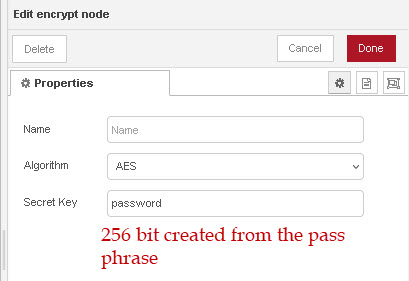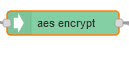By contrast the IV and Key need to be passed into the aes encrypt node(crypto-utils package).

This is done using a preceding function node as shown below:

```let data={};
data.key="ZZZZZZZZZZZZZZZZZZZZZZZZzzzzzzzz";
data.initVector="BBBBBBBBBBBBBBBB";

data.data=Buffer.from("this is a test messsage");
return msg;
```

The flow below will encrypt and decrypt a message using the encrypt and decrypt nodes, and the only configuration needed is the encryption algorithm(AES) and the pass phrase.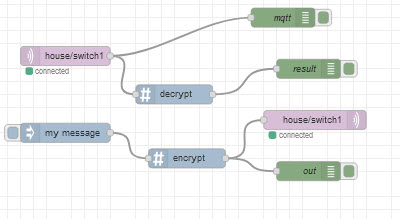When using the AES encrypt and AES decrypt nodes from the crypto-utils package we need to use a function to pass in the IV and key.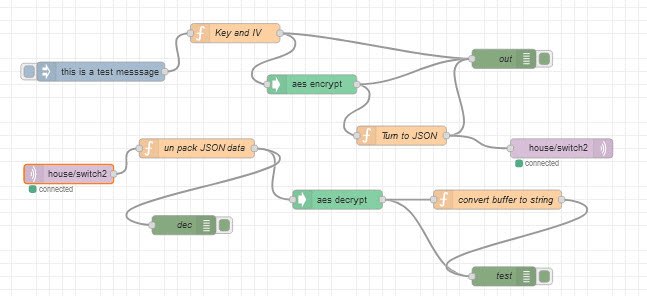The function node code for the encrypt node is shown below:

```let payload={};

return msg;
```

Note : In the above function the IV is fixed but in practise it needs to change and so it is normally random.

A common method is to use random bytes function as shown below:

```const initVector = crypto.randomBytes(16);
```

On the receive side we need to know the key and IV that were used to do the encryption.

The key is usually sent by some other method e.g.email but the IV is sent along with the encrypted data.

A common technique is to prepend or append it to the encrypted data as it can be stripped as we know that it is 16 bytes in length.

Another common method is to use JSON data, and to do this the binary encrypted data and IV needs to be either base64 encoded or hex encoded as it needs to be simple ASCII text type data.

Example code is shown below:

```let payload=msg.payload;

return msg;
```

### Manual Coding

For completeness we will also cover manual coding using the function node.

Although it is unlikely to be the preferred choice studying this method it is a very good way of of understanding the encryption/decryption process.

Here is the code for the function node to encrypt the data

```//const iv ="BBBBBBBBBBBBBBBB";
const key="ZZZZZZZZZZZZZZZZZZZZZZZZzzzzzzzz";
const algorithm = 'aes-256-ctr';
const IV_LENGTH = 16;
let iv = crypto.randomBytes(IV_LENGTH);
function encrypt(text) {

let cipher = crypto.createCipheriv(algorithm, key, iv);
let encrypted = cipher.update(text);
encrypted = Buffer.concat([encrypted, cipher.final()]);

// This returns a string
return iv.toString('hex') + ':' + encrypted.toString('hex');
// This returns a Json object
//let temp={"iv":iv.toString('hex'),"data":encrypted.toString('hex')};

}

return msg;
```

Firstly notice that I don’t need to use the global variable to access the crypto object as I am using mode-red v2 and this allows me to set this in the function node setup as shown below: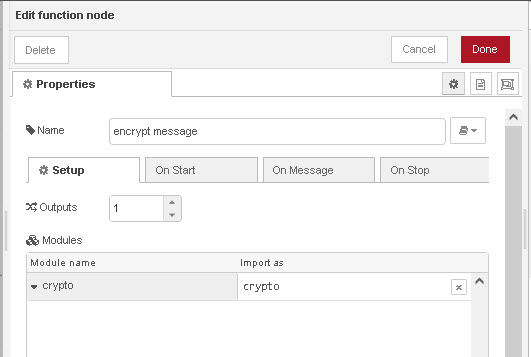Now the IV and key can be created manually as all we need is 16 ASCII characters for the IV and 32 for the key.

This is not a good strategy from a security viewpoint but it works and you see it often in examples on the web.

In the code above I use a manual key but a random IV

let iv = crypto.randomBytes(IV_LENGTH);//IV_LENGTH=16

Note that this produces a buffer object.

You could also use a random number for key just like with the IV but 32 bytes in length.

Here is  example code taken from here.

``const Securitykey = crypto.randomBytes(32);``

A pass phrase can also be use but you need to convert this into a key using SHA-256 for example.

Th encrypt function uses the crypto.createCipheriv() method as the crypto.createCipher() as been deprecated.

The interesting part is that it returns a string with the IV and encrypted data separated by a : (colon) character.

In the decrypt function we just split the string based on this character.

Alternatively we could place the IV and data in an object and thenwe would need to JSON encode the object before sending. The code is shown in the function but commented out.

The decrypt function is shown below:

```function decrypt(text) {
let textParts = text.split(':');
node.log(textParts);
let iv = Buffer.from(textParts, 'hex');
node.log("iv ="+iv);

let encryptedText = Buffer.from(textParts, 'hex');
let decipher = crypto.createDecipheriv(algorithm,key, iv);
let decrypted = decipher.update(encryptedText);
decrypted = Buffer.concat([decrypted, decipher.final()]);
return decrypted.toString();
}
```

The first part of the function extracts the IV and encrypted data before calling the decrypt method.

Notice both encrypt an decrypt have two calls update and final which must be called in order.

### Key Exchange

The encryption key and decryption key are the same as AES uses symmetrical encryption.

Therefore the key needs to be shared. There are a variety of  techniques used to do this securely see here.

In most case sensors are programmed with the key when deployed just like what happens on your home Wi-Fi network.

### Summary

There are many encryption nodes that you can use in node-red. The most popular and probably the easiest to use is the crypto node package.

You can also create you own function nodes but this is not really recommended unless you need some functionality not available in the crypto package.

Sending encrypted MQTT payloads will ensure that the data is encrypted all the way to the destination and doesn’t require any broker setup which means the data could be sent over public brokers.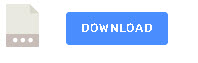Related Resources and Tutorials

Click to rate this post!
[Total: 0 Average: 0]

1.Stefan Denninger says:

Hi Steve, i need your help 😉

I am receiving data from my SmartMeter in Austria. The data is encrypted and I have got the key from my GridProvider.
Now the data is received by that python script: https://github.com/tosog/nbsm
but i am not able to port that to node-red ;(
can you help?
I think many people are searching for that solutions in my country.

i can send you examples of data.

1.steve says:

Stefan
Are those lines from the python script on github.
Are you rewriting the code into Javascript or using the python script?
Rgds
Steve

2.steve says:

I looked at the python code. Just so I’m clear the script takes data from an optical reader and sends it via MQTT as encrypted data and you want to get that data into node-red? IS that correct?
Rgds
Steve

1.Stefan Denninger says:

Wow, so fast answering 😉 Cool:
Yes, i want to port the whole script to node-red. Because i have two IR-Receivers, one is IR-USBserial connected and anotherone is sending ESP01-based over Wifi the data already to nodered and just for decryption the the python script (over mqtt).
I can send you all sources.

let data = {};
data.key = Buffer.from(“4D635XXX7782141”, ‘hex’);
let systitle = buf.slice(14, 22); // 8 bytes
let nonce = buf.slice(24, 28); // 4 bytes
let initvec = systitle + nonce
data.initVector = Buffer.from(initvec);
data.data = buf.slice(28, 108);
return msg;
-> sending to #decrypt from crypto-js but algorithm is aes-128-gcm, so not working.
———————– second try – discrete ———————————
let data = {};
const algorithm = ‘aes-128-gcm’;
const key = “4D635…..”;

var systitle = buf.slice(14, 22); // 8 bytes
var nonce = buf.slice(24, 28); // 4 bytes
var initvec = systitle + nonce
var iv = Buffer.from(initvec).toString(‘hex’);
var encdata = buf.slice(28, 108).toString(‘hex’);

function decrypt(text) {
let textParts = text.split(‘:’);
node.log(textParts);
let iv = Buffer.from(textParts, ‘hex’);
node.log(“iv =”+iv);

let encryptedText = Buffer.from(textParts, ‘hex’);
let decipher = crypto.createDecipheriv(algorithm,key, iv);
let decrypted = decipher.update(encryptedText);
decrypted = Buffer.concat([decrypted, decipher.final()]);
return decrypted.toString();
}
1.steve says: